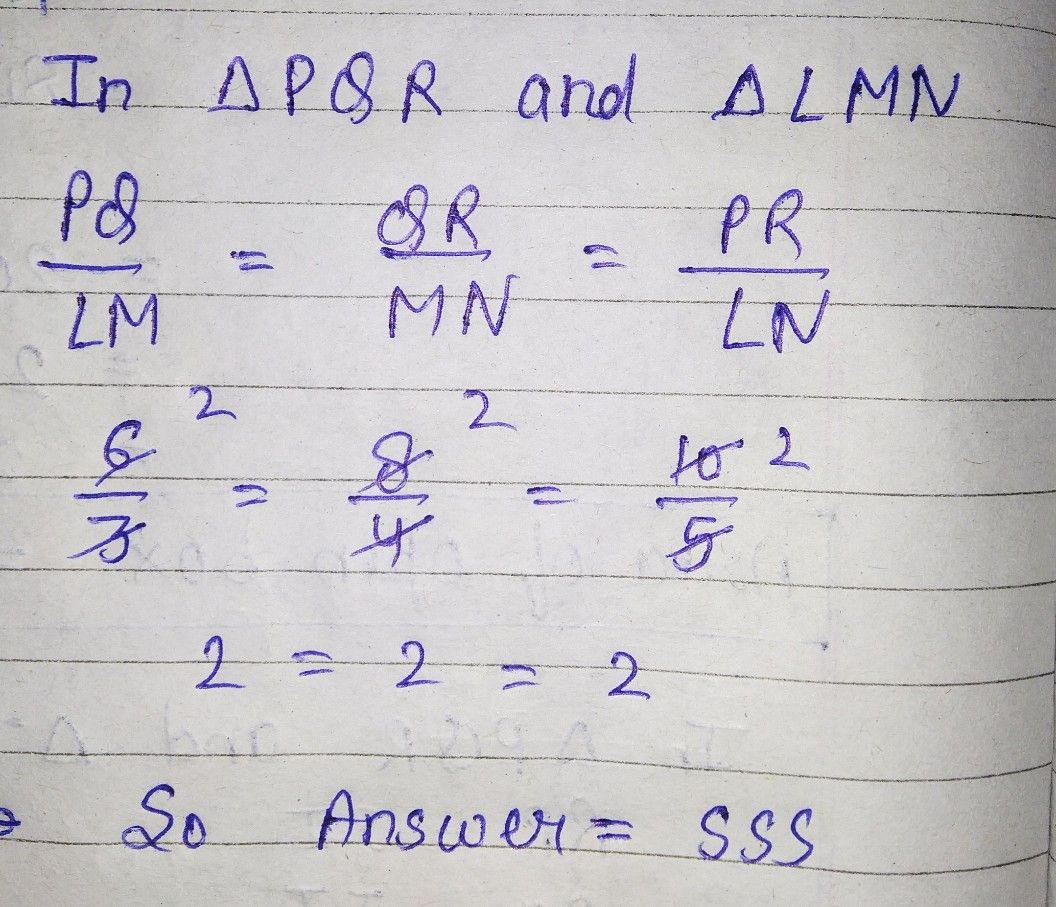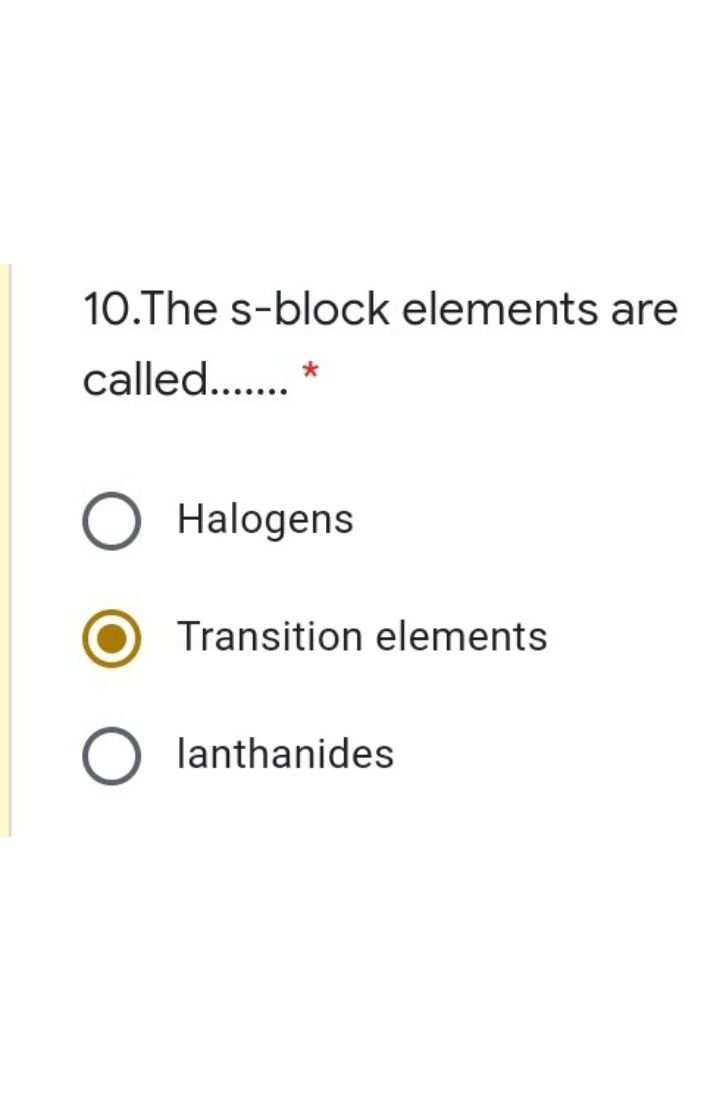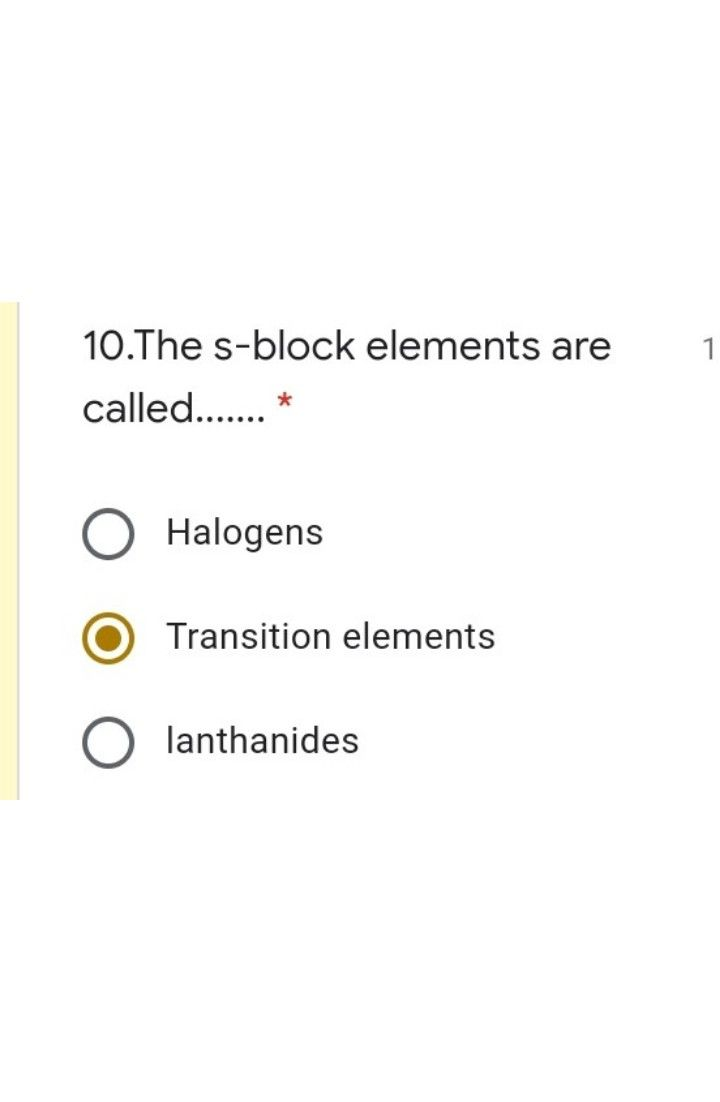Symbol
Problem$P$ Are the triangles in figure $si$ similar? If yes, by which test ? $10$ $L$ $6$ $3$ $5$ $Q$ $8$ $R$ $M$ $4$ $N$ $○$ $SAS$ $SSS$ $\right)$ O $AAS$ O Not Similar
10th-13th grade
Geometry
Search count: 107
SolutionQanda teacher - AnkitaIf you have any doubts please do let me know dear. if you are satisfied with my answer please do LIKE and give a 5 STAR RATING and coins as THANK U GIFT..! ALL THE BEST..!!!?Studentsolve the question